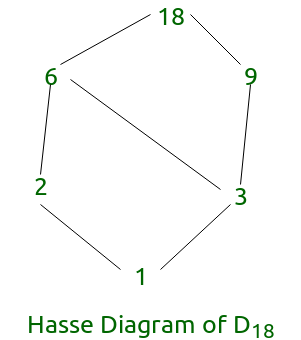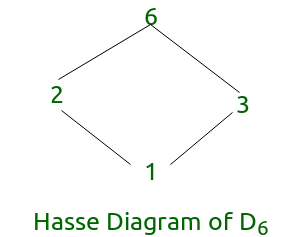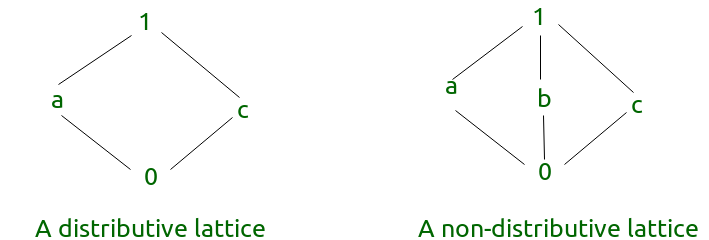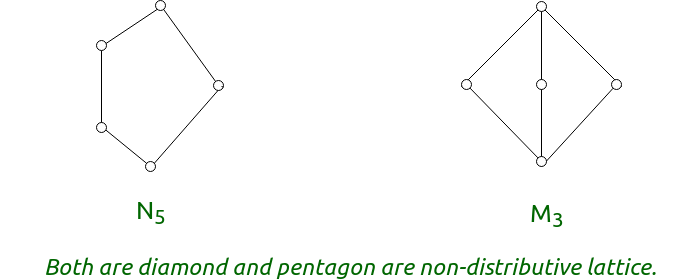# Partial Orders and Lattices (Set-2) | Mathematics

Prerequisite: Partial Orders and Lattices | Set-1

Well Ordered Set –
Given a poset, (X, ≤) we say that ≤ is a well-order (well-ordering) and that is well-ordered by ≤ iff every nonempty subset of X has a least element. When X is non-empty, if we pick any two-element subset, {a, b}, of X, since the subset {a, b} must have a least element, we see that either a≤b or b≤a, i.e., every well-order is a total order.
E.g. – The set of natural number (N) is a well ordered.

Bounded Lattice:
A lattice L is said to be bounded if it has the greatest element I and a least element 0.
E.g. – D18= {1, 2, 3, 6, 9, 18} is a bounded lattice.Note: Every Finite lattice is always bounded.

Complemented Lattice:
A lattice L is said to be complemented if it is bounded and if every element in L has a complement. Here, each element should have atleast one complement.
E.g. – D6 {1, 2, 3, 6} is a complemented lattice.In the above diagram every element has a complement.

Distributive Lattice:
It is a lattice in which meet (∧) and join (∨) operations distribute over each other.

• x ∧ (y ∨ z) = (x ∧ y) ∨ (x ∧ z)
• x ∨ (y ∧ z) = (x ∨ y) ∧ (x ∨ z)Note:

• A complemented distributive lattice is a boolean algebra or boolean lattice.
• A lattice is distributive if and only if none of its sublattices is isomorphic to N5 or M3.
• For distributive lattice each element has unique complement. This can be used as a theorm to prove that a lattice is not distributive.

My Personal Notes arrow_drop_upCheck out this Author's contributed articles.

If you like GeeksforGeeks and would like to contribute, you can also write an article using contribute.geeksforgeeks.org or mail your article to contribute@geeksforgeeks.org. See your article appearing on the GeeksforGeeks main page and help other Geeks.

Please Improve this article if you find anything incorrect by clicking on the "Improve Article" button below.

Article Tags :

Be the First to upvote.

Please write to us at contribute@geeksforgeeks.org to report any issue with the above content.